## How to Calculate and Solve for Inter-atomic Spacing | Bragg’s Law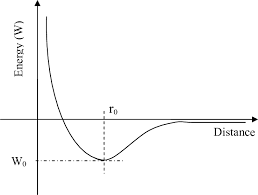The image above represents inter-atomic spacing.

To compute for inter-atomic spacing, three essential parameters are needed and these parameters are Order of Reflection (n), Wavelength (λ) and Angle of Diffraction (θ).

The formula for calculating inter-atomic spacing:

d = /2sinθ

Where:

d = Inter-atomic Spacing
λ = Wavelength
n = Order of Reflection
θ = Angle of Diffraction

Let’s solve an example;
Find the inter-atomic spacing when the wavelength is 12, order of reflection is 4 and the angle of diffraction is 6.

This implies that;

λ = Wavelength = 12
n = Order of Reflection = 4
θ = Angle of Diffraction = 6

d = /2sinθ
d = (12)(4)/2sin(6°)
d = 48/2(0.104)
d = 48/0.209
d = 229.6

Therefore, the inter-atomic spacing is 229.6 m.

## How to Calculate and Solve for Conversion of Volume Fraction to Mass Fraction | Phase Transformation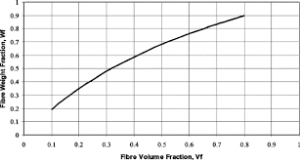The image above represents the conversion of volume fraction to mass fraction.

To compute for volume fraction to mass fraction, four essential parameters are needed and these parameters are α-phase Volume Fraction (Vα), β-phase Volume Fraction (Vβ), α-phase Density (ρα) and β-phase Density (ρβ).

The formula for calculating volume fraction to mass fraction:

Wα = Vαρα/(Vαρα) + (Vβρβ)

Wβ = Vβρβ/(Vαρα) + (Vβρβ)

Where:

Wα = α-phase Weight/Mass Fraction
Wβ = β-phase Weight/Mass Fraction
Vα = α-phase Volume Fraction
Vβ = β-phase Volume Fraction
ρα = α-phase Density
ρβ = β-phase Density

Let’s solve an example;
Find the conversion of volume fraction to mass fraction when the α-phase volume fraction is 4, the β-phase volume fraction is 7, the α-phase density is 11 and the β-phase density is 10.

This implies that;

Vα = α-phase Volume Fraction = 4
Vβ = β-phase Volume Fraction = 7
ρα = α-phase Density = 11
ρβ = β-phase Density = 10

Wα = (4)(11)/((4)(11)) + ((7)(10))
Wα = (44)/(44) + (70)
Wα = (44)/(114)
Wα = 0.38

Therefore, the α-phase mass fraction, Wα is 0.38.

Wβ = (7)(10)/((4)(11)) + ((7)(10))
Wβ = (70)/(44) + (70)
Wβ = (70)/(114)
Wβ = 0.614

Therefore, the β-phase mass fraction, Wβ is 0.614.

## How to Calculate and Solve for Net Force between Two Atoms | Crystal Structures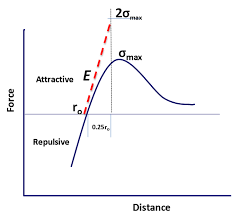The image above represents net force between two atoms.

To compute for net force between two atoms, two essential parameters are needed and these parameters are Attractive Force (FAand Repulsive Force (FR).

The formula for calculating net force between two atoms:

FN = FA + FR

Where:

FN = Net Force between Two Atoms
FA = Attractive Force
FR = Repulsive Force

Given an example;
Find the net force between two atoms when the attractive force is 15 and the repulsive force is 3.

This implies that;

FA = Attractive Force = 15
FR = Repulsive Force = 3

FN = FA + FR
FN = 15 + 3
FN = 18

Therefore, the net force between two atoms is 18 N.

## How to Calculate and Solve for Planar Density | Crystal Structures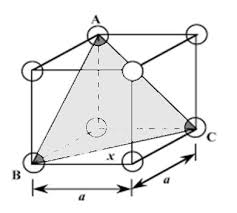The image above represents planar density.

To compute for planar density, two essential parameters are needed and these parameters are Number of Atoms Centered on the Plane (N) and Area of the Plane (A).

The formula for calculating planar density:

PD = N/A

Where:

PD = Planar Density
N = Number of Atoms Centered on the Plane
A = Area of the Plane

Given an example;
Find the planar density when the number of atoms centered on the plane is 24 and the area of the plane is 3.

This implies that;

N = Number of Atoms Centered on the Plane = 24
A = Area of the Plane = 3

PD = N/A
PD = 24/3
PD = 8

Therefore, the planar density is 8 atoms/m².

## How to Calculate and Solve for Linear Density | Crystal Structures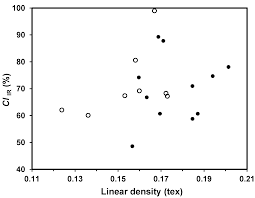The image above represents linear density.

To compute for linear density, two essential parameters are needed and these parameters are Number of Atoms Centered on Direction Vector (N) and Length of Direction Vector (L).

The formula for calculating linear density:

LD = N/L

Where:

LD = Linear Density
N = Number of Atoms Centered on Direction Vector
L = Length of Direction Vector

Given an example;
Find the linear density when the number of atoms centered on direction vector is 10 and the length of direction vector is 2.

This implies that;

N = Number of Atoms Centered on Direction Vector = 10
L = Length of Direction Vector = 2

LD = N/L
LD = 10/2
LD = 5

Therefore, the linear density is 5 atoms/m.

## How to Calculate and Solve for Hexagonal Crystals | Crystal Structures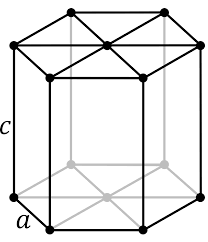The image above represents hexagonal crystals.

To compute for hexagonal crystals, two essential parameters are needed and these parameters are Miller Index (h) and Miller Index (k).

The formula for calculating hexagonal crystals:

i = -(h + k)

Where:

i = Hexagonal Crystals
h = Miller Index
k = Miller Index

Given an example;
Find the hexagonal crystals when the miller index is 22 and the miller index is 11.

This implies that;

h = Miller Index = 22
k = Miller Index = 11

i = -(h + k)
i = -(22 + 11)
i = -(33)
i = -33

Therefore, the hexagonal crystals is -33.

## How to Calculate and Solve for Theoretical Density of Metals | Crystal Structures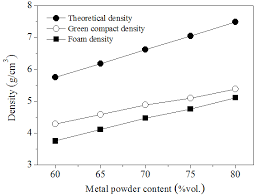The image above represents theoretical density of metals.

To compute for theoretical density of metals, four essential parameters are needed and these parameters are Number of Atoms Associated in the Cell (n), Atomic Weight (A), Volume of Unit Cell (Vcand Avogadro’s Number (NA).

The formula for calculating theoretical density of metals:

ρ = nA/VcNA

Where:

ρ = Theoretical Denity of the Metal
n = Number of Atoms Associated in the Cell
A = Atomic Weight
Vc = Volume of Unit Cell

Let’s solve an example;
Find the theoretical density of the metal when the number of atoms associated in the cell is 3, the atomic weight is 6, the volume of unit cell is 2 and the avogadro’s number is 6.022e+24.

This implies that;

n = Number of Atoms Associated in the Cell = 3
A = Atomic Weight = 6
Vc = Volume of Unit Cell = 2
NA = Avogadro’s Number = 6.022e+24

ρ = nA/VcNA
ρ = (3)(6)/(2)(6.0221e+23)
ρ = (18)/(1.20442e+24)
ρ = 1.49

Therefore, the theoretical density of metals is 1.49 m.

## How to Calculate and Solve for Unit Cell Edge Length | Crystal Structures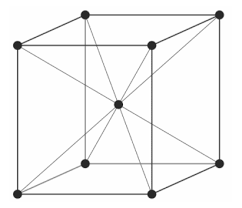The image above represents unit cell edge length.

To compute for unit cell edge length, one essential parameter is needed and this parameter is Radius of an Atom (R).

The formula for calculating unit cell edge length:

a = 4R/√(3)

Where:

a = Unit Cell Edge Length, BCC
R = Radius of the atom

Given an example;
Find the unit cell edge length when the radius of the atom is 6.

This implies that;

R = Radius of the atom = 6

a = 4R/√(3)
a = 4(6)/√(3)
a = 24/1.73
a = 13.85

Therefore, the unit cell edge length is 13.85 m.

## How to Calculate and Solve for Atomic Packing Factor | Crystal Structures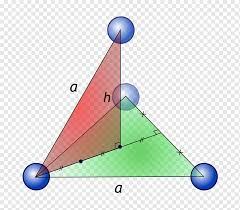The image above represents atomic packing factor.

To compute for atomic packing factor, two essential parameters are needed and these parameters are Volume of Atoms in a Unit Cell (V) and Total Unit Cell Volume (T).

The formula for calculating atomic packing factor:

APF = V/T

Where:

APF = Atomic Packing Factor
V = Volume of Atoms in a Unit Cell
T = Total Unit Cell Volume

Let’s solve an example;
Find the atomic packing factor when the volume of atoms in a unit cell is 6 and the total unit cell volume is 3.

This implies that;

V = Volume of Atoms in a Unit Cell = 6
T = Total Unit Cell Volume = 3

APF = V/T
APF = 6/3
APF = 2

Therefore, the atomic packing factor is 2.

## How to Calculate and Solve for Unit Cell Edge Length | Crystal Structures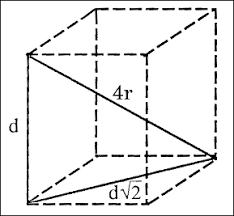The image above represents unit cell edge length.

To compute for unit cell edge length, one essential parameter is needed and this parameter is Radius of an Atom (R).

The formula for calculating unit cell edge length:

a = 2R√(2)

Where:

a = Unit Cell Edge Length, FCC
R = Radius of the Atom

Given an example;
Find the unit cell edge length when the radius of the atom is 12.

This implies that;

R = Radius of the Atom = 12

a = 2R√(2)
a = 2(12)√(2)
a = (24)(1.41)
a = 33.94

Therefore, the unit cell edge length is 33.94 m.